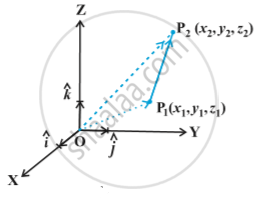# Vector Joining Two Points

#### notes

If P_1(x_1, y_1, z_1) and P_2(x_2, y_2, z_2) are any two points, then the vector joining P_1 and P_2 is the vector  in following fig.Joining the points P_1 and P_2 with the origin O, and applying triangle law, from the triangle OP_1P_2, we have
vec (OP_1) + vec (P_1P_2) = vec (OP_2)
Using the properties of vector addition, the above equation becomes
vec (P_1P_2) = vec (OP_2) - vec (OP_1)
i.e. vec (P_1P_2) = (x_2hat i + y_2hatj + z_2hat k) - (x_1hati + y_1hatj + z_1 hat k)
= (x_2 - x_1)hat i + (y_2 - y_1)hat j + (z_2 -z_1) hat k

The magnitude of vector vec(P_1P_2) is given by
|vec (P_1P_2)| = sqrt ((x_2 -x_1)^2  + (y_2 - y_1)^2 + (z_2-z_1)^2)

If you would like to contribute notes or other learning material, please submit them using the button below.

### Shaalaa.com

Vector Algebra part 12 (Vector joining 2 points) [00:10:47]
S
0%